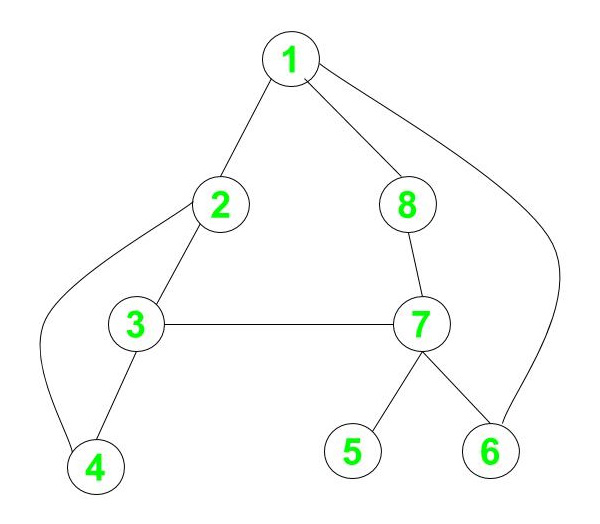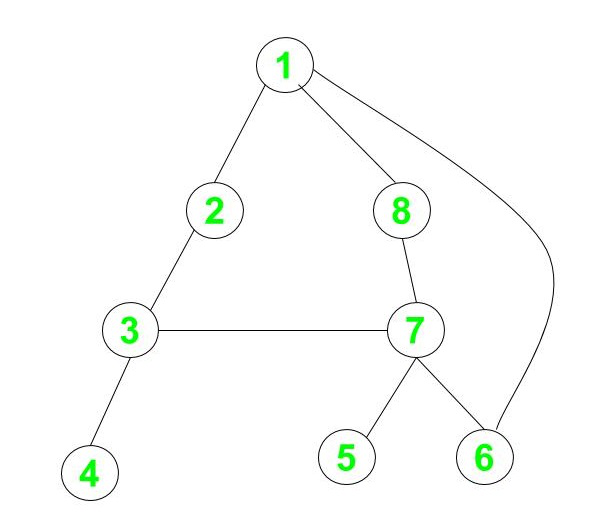# Check if a given Graph is 2-edge connected or not

Given an undirected graph G, with V vertices and E edges, the task is to check whether the graph is 2-edge connected or not.

A graph is said to be 2-edge connected if, on removing any edge of the graph, it still remains connected, i.e. it contains no Bridges.

Examples:

Input: V = 8, E = 10Output: Yes
Explanation:
Given any vertex in the graph, we can reach any other vertex in the graph. Moreover, removing any edge from the graph does not affect its connectivity. So, the graph is said to be 2-edge connected.

Input: V = 8, E = 9Output: No
Explanation:
On removal of the edge between vertex 3 and vertex 4, the graph is not connected anymore. So, the graph is not 2-edge connected.

## Recommended: Please try your approach on {IDE} first, before moving on to the solution.

Naive Approach: The naive approach is to check that on removing any edge X, if the remaining graph G – X is connected or not. If the graph remains connected on removing every edge one by one then it is a 2-edge connected graph. To implement the above idea, remove an edge and perform Depth First Search(DFS) or Breadth-First Search(BFS) from any vertex and check if all vertices are covered or not. Repeat this process for all E edges. If all vertices cannot be traversed for any edge, print No. Otherwise, print Yes.
Time Complexity: O(E * ( V + E))
Auxiliary Space: O(1)

Efficient Approach: Since the given graph is undirected, the problem can be solved only by counting the number of edges connected to the nodes. If for any of the nodes, the number of edges connected to it is 1 it means on removing this edge the node becomes disconnected and it can’t be reached from any other node therefore the graph is not 2-edge connected. Below are the steps:

1. Create an array noOfEdges[] of size V which will store the number of edges connected to a node.
2. For every edge (u, v) increment the number of edges for node u and v.
3. Now iterate over the array noOfEdges[] and check if any of the edge has only 1 edge connected to it. If yes then the graph is not 2-edge connected.
4. Otherwise the graph is 2-edge connected.

Below is the implementation of the above approach:

## C++14

 `// C++14 program for the above approach ` ` `  `#include ` `using` `namespace` `std; ` ` `  `// Definition of a graph ` `class` `Graph { ` ` `  `    ``// No. of vertices ` `    ``int` `V; ` ` `  `    ``// To create adjacency list ` `    ``list<``int``>* adj; ` ` `  `public``: ` `    ``// Constructor ` `    ``Graph(``int` `V); ` ` `  `    ``// Function to add an edge to graph ` `    ``void` `addEdge(``int` `v, ``int` `w); ` ` `  `    ``// Function to check 2-edge ` `    ``// 2-edge connectivity ` `    ``void` `twoEdge(``int` `v); ` `}; ` ` `  `// Initialize the graph ` `Graph::Graph(``int` `V) ` `{ ` `    ``this``->V = V; ` `    ``adj = ``new` `list<``int``>[V]; ` `} ` ` `  `// Adding edges to adjacency list ` `void` `Graph::addEdge(``int` `v, ``int` `w) ` `{ ` `    ``adj[v - 1].push_back(w - 1); ` `    ``adj[w - 1].push_back(v - 1); ` `} ` ` `  `// Function to find if the graph is ` `// 2 edge connected or not ` `void` `Graph::twoEdge(``int` `v) ` `{ ` `    ``// To store number of edges for ` `    ``// each node ` `    ``int` `noOfEdges[v]; ` ` `  `    ``for` `(``int` `i = 0; i < v; i++) { ` `        ``noOfEdges[i] = adj[i].size(); ` `    ``} ` ` `  `    ``bool` `flag = ``true``; ` ` `  `    ``// Check the number of edges ` `    ``// connected to each node ` `    ``for` `(``int` `i = 0; i < v; i++) { ` ` `  `        ``if` `(noOfEdges[i] < 2) { ` `            ``flag = ``false``; ` `            ``break``; ` `        ``} ` `    ``} ` ` `  `    ``// Print the result ` `    ``if` `(flag) ` `        ``cout << ``"Yes"``; ` `    ``else` `        ``cout << ``"No"``; ` `} ` ` `  `// Driver Code ` `int` `main() ` `{ ` `    ``// Number of nodes and edges ` `    ``int` `V = 8; ` `    ``int` `E = 10; ` ` `  `    ``// Given Edges ` `    ``int` `edges[E] = { { 1, 2 }, { 1, 8 }, { 1, 6 },  ` `                        ``{ 2, 3 }, { 2, 4 }, { 3, 7 },  ` `                        ``{ 3, 4 }, { 7, 5 }, { 7, 6 },  ` `                        ``{ 7, 8 } }; ` ` `  `    ``// Initialize the graph ` `    ``Graph g(V); ` ` `  `    ``// Adding the edges to graph ` `    ``for` `(``int` `i = 0; i < E; i++) { ` `        ``g.addEdge(edges[i], edges[i]); ` `    ``} ` ` `  `    ``// Function call ` `    ``g.twoEdge(V); ` ` `  `    ``return` `0; ` `} `

## Java

 `// Java program for the above approach ` `import` `java.util.*; ` ` `  `class` `Graph{ ` `     `  `// No. of vertices      ` `private` `int` `V;  ` ` `  `// Array of lists for Adjacency ` `// List Representation  ` `private` `LinkedList adj[];  ` ` `  `// Constructor  ` `@SuppressWarnings``(``"unchecked"``) ` `Graph(``int` `v)  ` `{  ` `    ``V = v;  ` `    ``adj = ``new` `LinkedList[v]; ` `     `  `    ``for``(``int` `i = ``0``; i < v; ++i)  ` `        ``adj[i] = ``new` `LinkedList();  ` `}  ` ` `  `// Function to add an edge into the graph  ` `void` `addEdge(``int` `v, ``int` `w)  ` `{  ` `    ``adj[v - ``1``].add(w - ``1``); ``// Add w to v's list.  ` `    ``adj[w - ``1``].add(v - ``1``); ` `}  ` ` `  `// Function to find if the graph is ` `// 2 edge connected or not ` `void` `twoEdge(``int` `v) ` `{ ` `     `  `    ``// To store number of edges for ` `    ``// each node ` `    ``int``[] noOfEdges = ``new` `int``[v]; ` `    ``for``(``int` `i = ``0``; i < v; i++)  ` `    ``{ ` `        ``noOfEdges[i] = adj[i].size(); ` `    ``} ` ` `  `    ``boolean` `flag = ``true``; ` `     `  `    ``// Check the number of edges ` `    ``// connected to each node ` `    ``for``(``int` `i = ``0``; i < v; i++) ` `    ``{ ` `        ``if` `(noOfEdges[i] < ``2``) ` `        ``{ ` `            ``flag = ``false``; ` `            ``break``; ` `        ``} ` `    ``} ` `     `  `    ``// Print the result ` `    ``if` `(flag) ` `        ``System.out.print(``"Yes"``); ` `    ``else` `        ``System.out.print(``"No"``); ` `} ` ` `  `// Driver code ` `public` `static` `void` `main (String[] args)  ` `{ ` `     `  `    ``// Number of nodes and edges ` `    ``int` `V = ``8``; ` `    ``int` `E = ``10``; ` `     `  `    ``// Given Edges ` `    ``int` `edges[][] = { { ``1``, ``2` `}, { ``1``, ``8` `}, ` `                      ``{ ``1``, ``6` `}, { ``2``, ``3` `}, ` `                      ``{ ``2``, ``4` `}, { ``3``, ``7` `},  ` `                      ``{ ``3``, ``4` `}, { ``7``, ``5` `},  ` `                      ``{ ``7``, ``6` `}, { ``7``, ``8` `} }; ` `     `  `    ``Graph g = ``new` `Graph(V);  ` `     `  `    ``// Adding the edges to graph ` `    ``for``(``int` `i = ``0``; i < E; i++)  ` `    ``{ ` `        ``g.addEdge(edges[i][``0``], edges[i][``1``]); ` `    ``} ` `     `  `    ``// Function call ` `    ``g.twoEdge(V); ` `} ` `} ` ` `  `// This code is contributed by offbeat `

Output:

```No
```

Time Complexity: O(V + E)
Auxiliary Space: O(V)

Attention reader! Don’t stop learning now. Get hold of all the important DSA concepts with the DSA Self Paced Course at a student-friendly price and become industry ready.

My Personal Notes arrow_drop_upCheck out this Author's contributed articles.

If you like GeeksforGeeks and would like to contribute, you can also write an article using contribute.geeksforgeeks.org or mail your article to contribute@geeksforgeeks.org. See your article appearing on the GeeksforGeeks main page and help other Geeks.

Please Improve this article if you find anything incorrect by clicking on the "Improve Article" button below.

Improved By : offbeat